# Wolfram ImageIdentify Net V1

Identify the main object in an image

Released in 2017 by Wolfram Research, this net was trained on over 4,000 classes of objects. It is part of the back end for the ImageIdentify function in Wolfram Language 11.1. It was designed to achieve a good balance among classification accuracy, size and evaluation speed.

Number of layers: 232 | Parameter count: 14,713,147 | Trained size: 65 MB |

## Training Set Information

• Internal Wolfram ImageIdentify training set, consisting of over 3 million training images and over 4,000 classes of objects (not publicly available).

## Examples

### Resource retrieval

Get the pre-trained net:

 In:=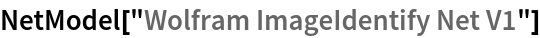Out=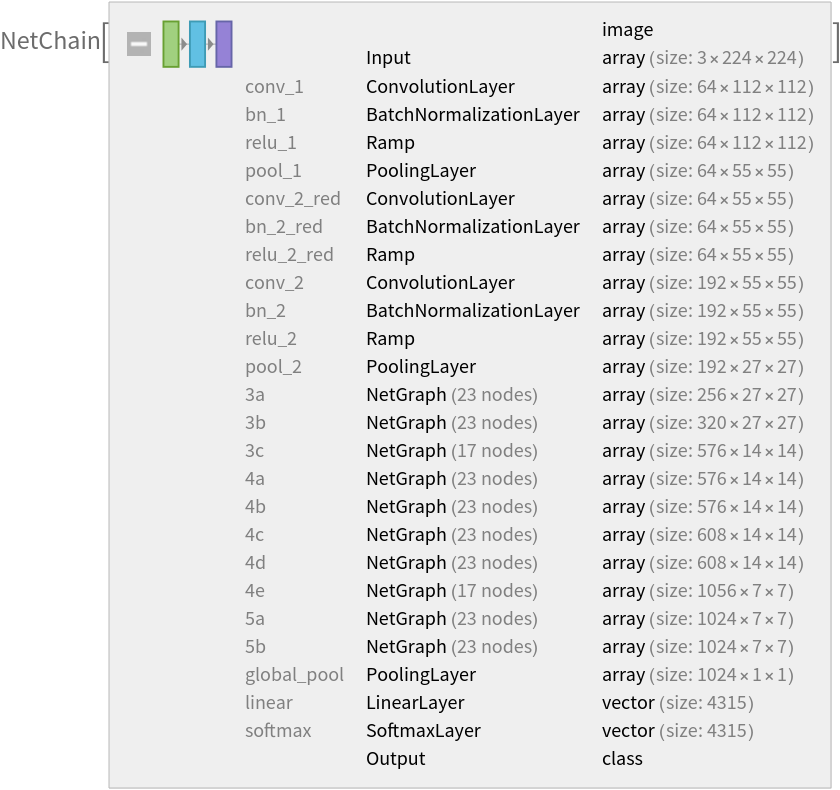### Basic usage

Classify an image:

 In:=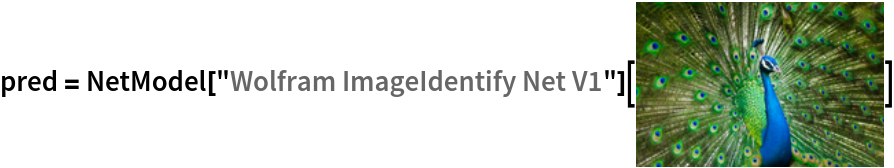Out=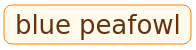The prediction is an Entity object, which can be queried:

 In:=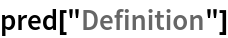Out=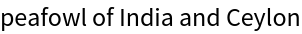Get a list of available properties of the predicted Entity:

 In:=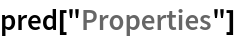Out=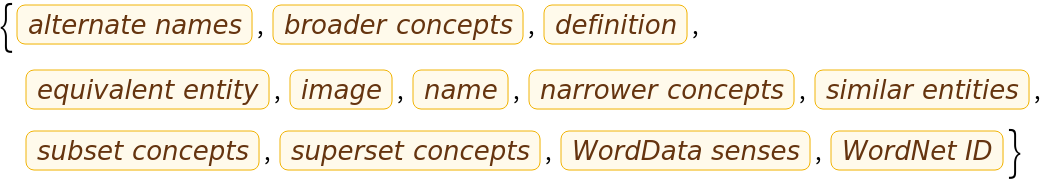Obtain the probabilities of the ten most likely entities predicted by the net:

 In:=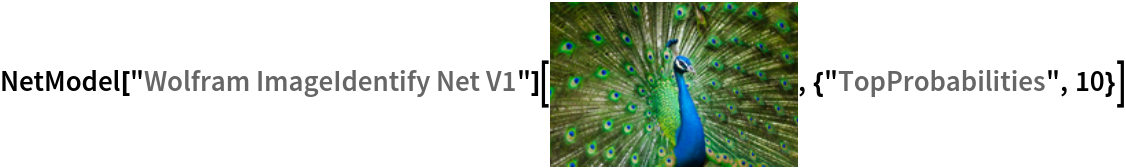Out=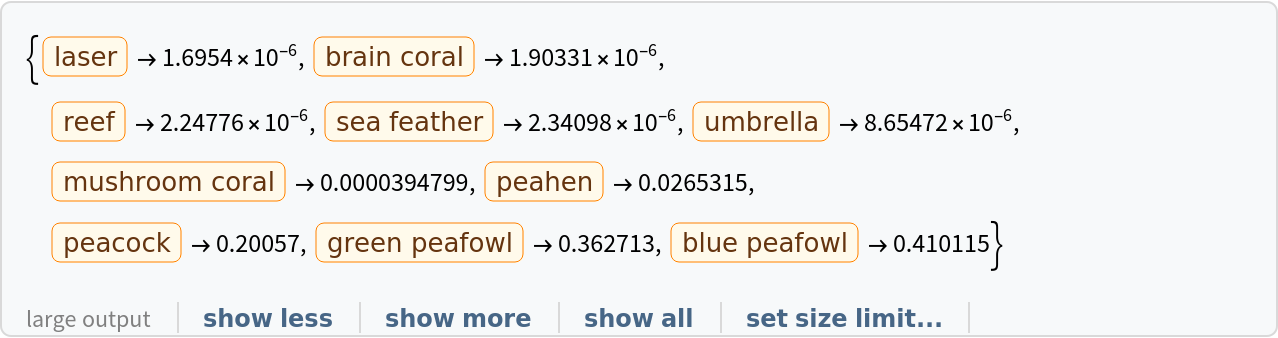An object outside the list of the ImageIdentify dataset will be misidentified:

 In:=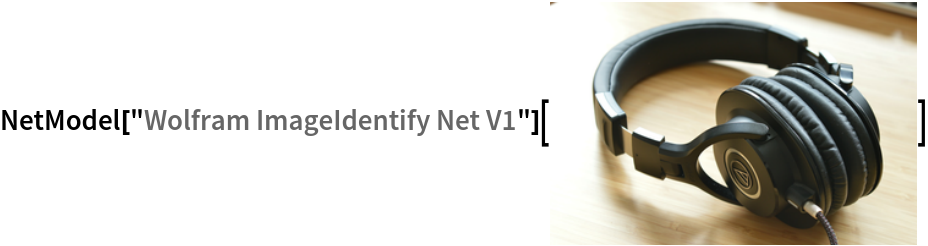Out=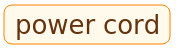Obtain the list of names of all available classes:

 In:=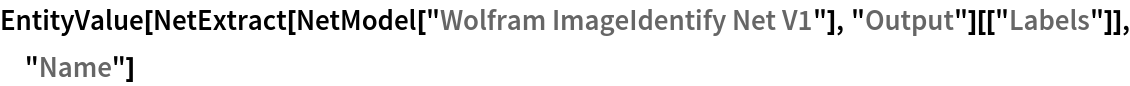Out=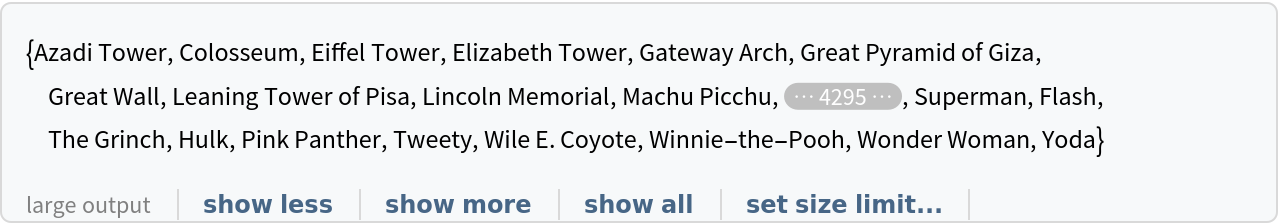### Feature extraction

Remove the last two layers of the trained net so that the net produces a vector representation of an image:

 In:=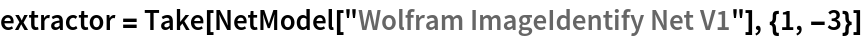Out=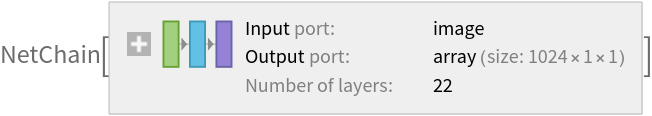Get a set of images:

 In:=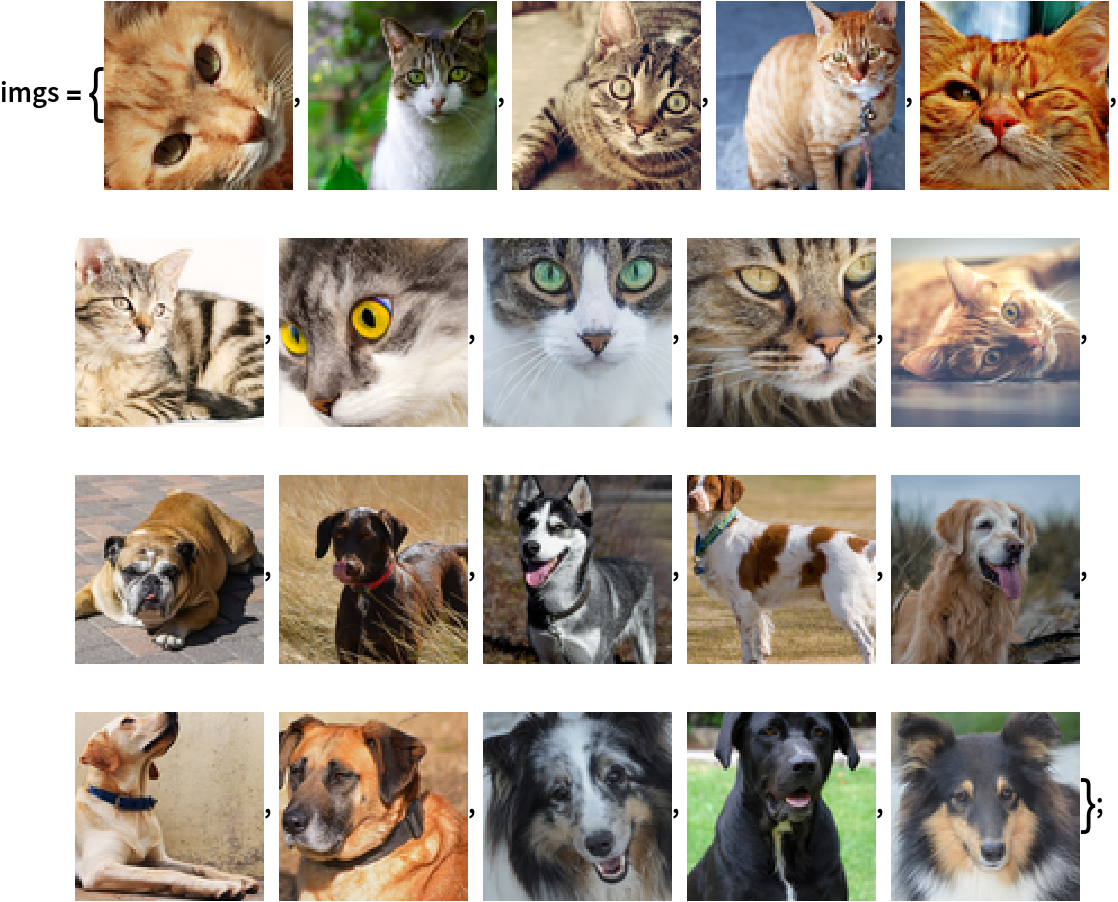Visualize the features of a set of images:

 In:=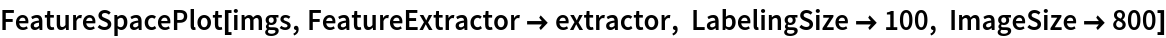Out=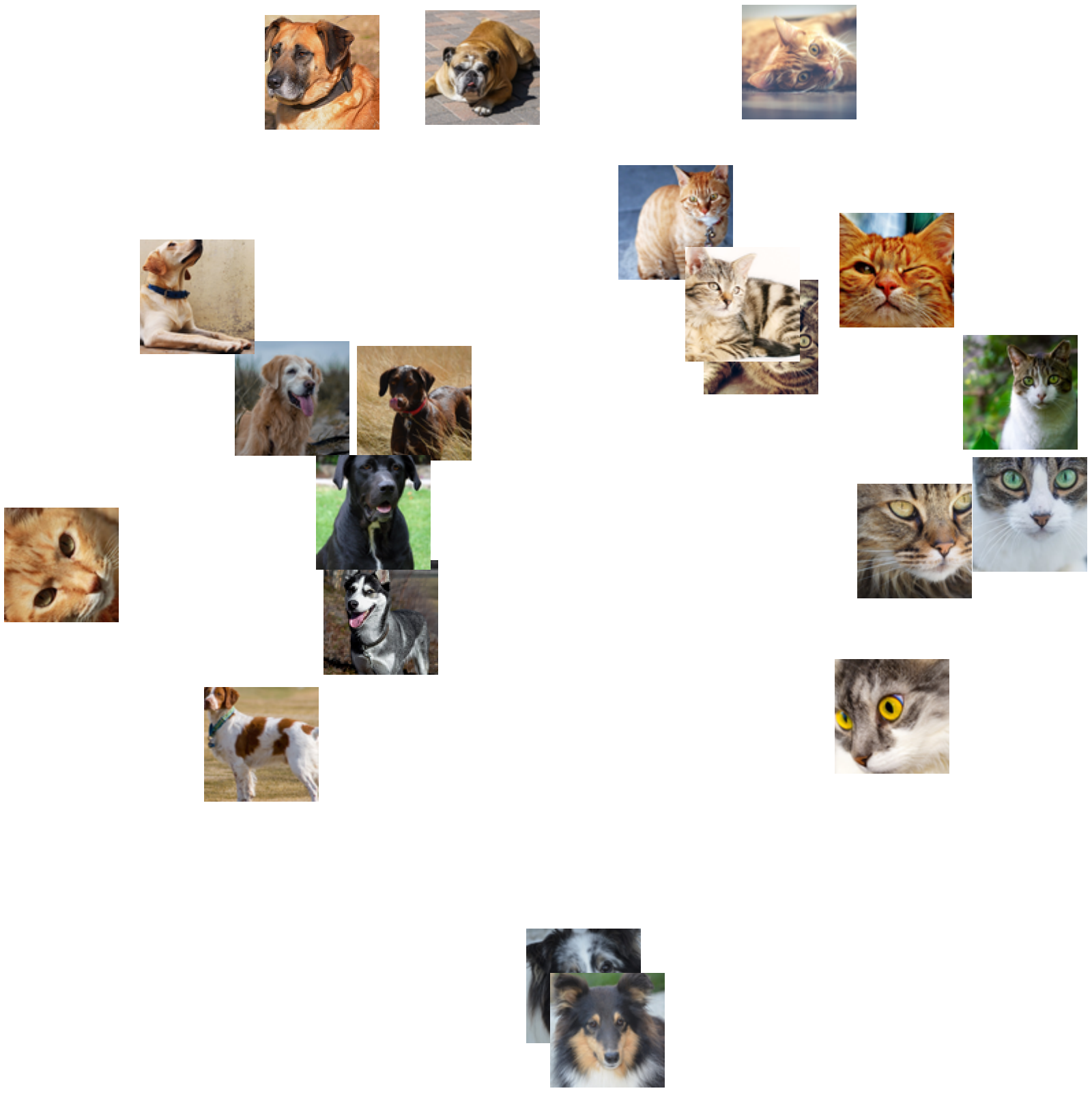### Visualize convolutional weights

Extract the weights of the first convolutional layer in the trained net:

 In:=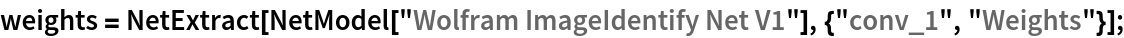Visualize the weights as a list of 64 images of size 7x7:

 In:=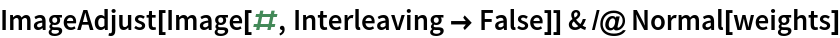Out=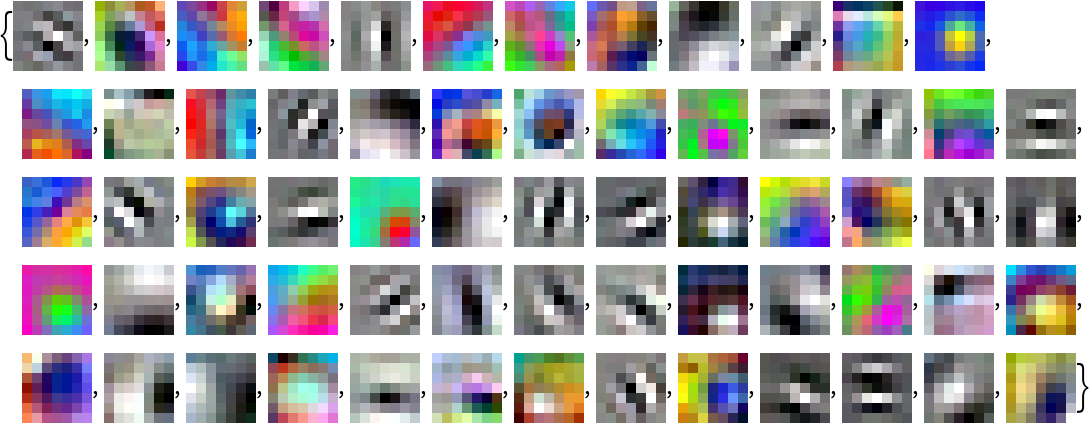### Transfer learning

Use the pre-trained model to build a classifier for telling apart images of dogs and cats. Create a test set and a training set:

 In:=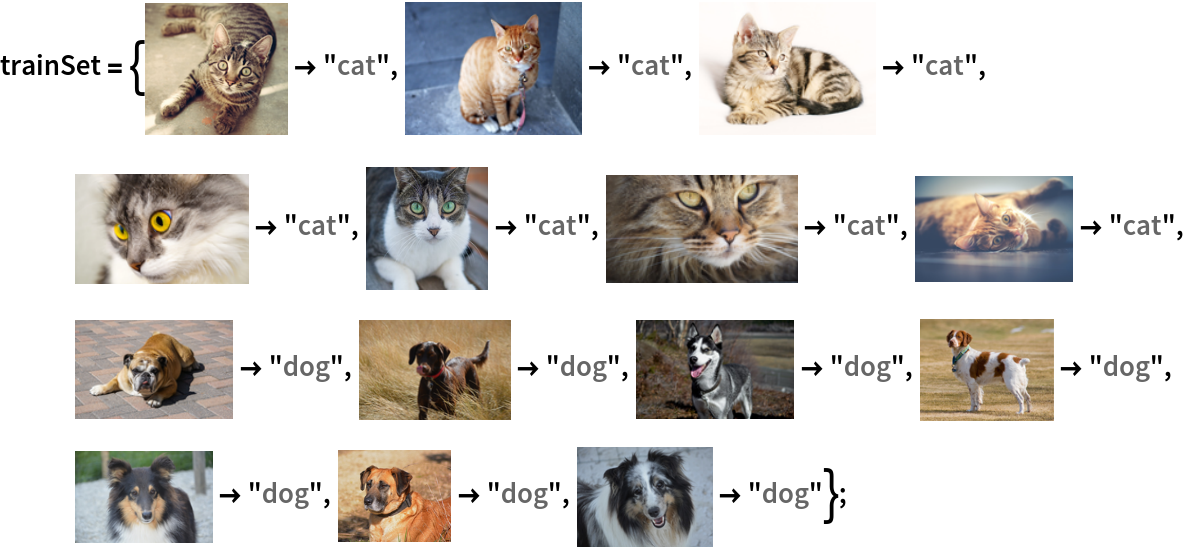In:=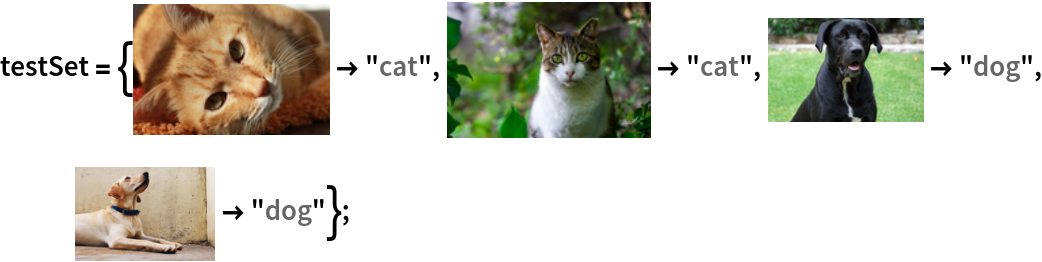Remove the linear layer from the pre-trained net:

 In:=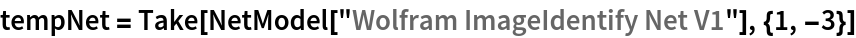Out=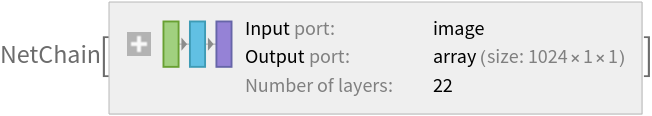Create a new net composed of the pre-trained net followed by a linear layer and a softmax layer:

 In:=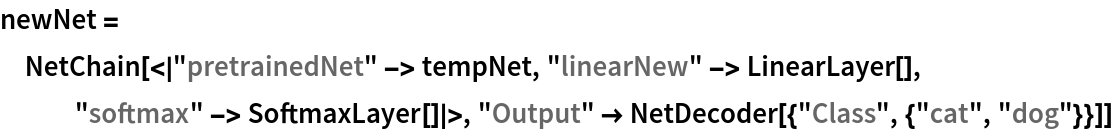Out=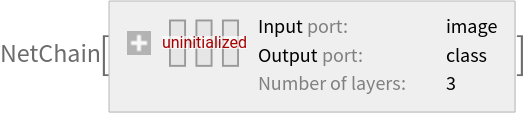Train on the dataset, freezing all the weights except for those in the "linearNew" layer (use TargetDevice -> "GPU" for training on a GPU):

 In:=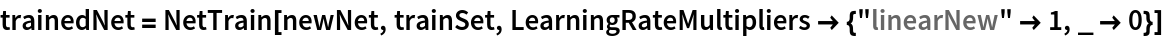Out=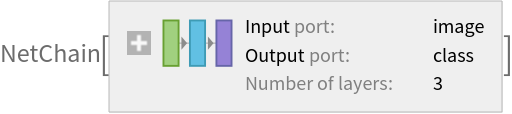Perfect accuracy is obtained on the test set:

 In:=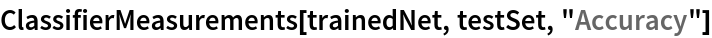Out=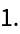### Net information

Inspect the number of parameters of all arrays in the net:

 In:=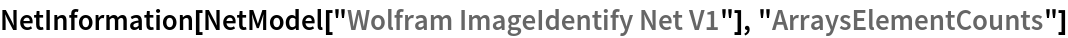Out=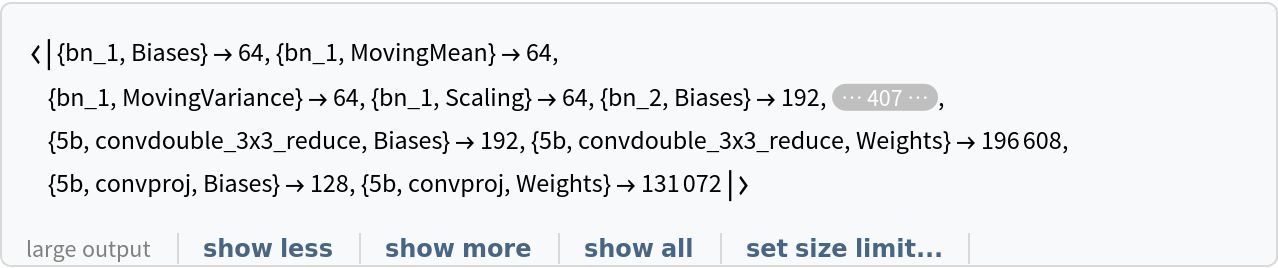Obtain the total number of parameters:

 In:=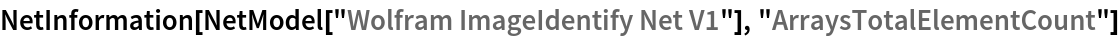Out=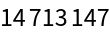Obtain the layer type counts:

 In:=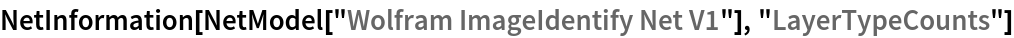Out=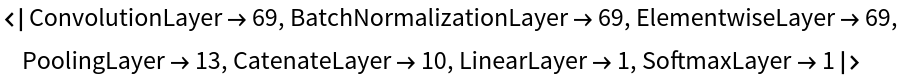Display the summary graphic:

 In:=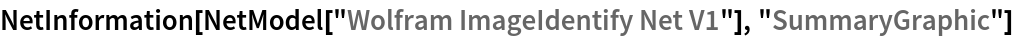Out=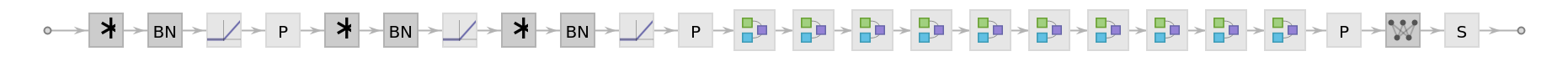### Export to MXNet

Export the net into a format that can be opened in MXNet :

 In:=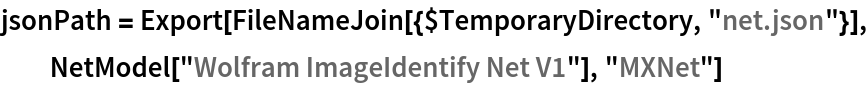Out=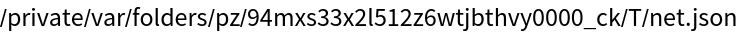Export also creates a net.params file containing parameters:

 In:=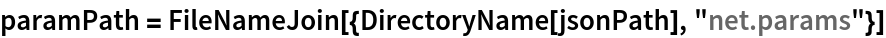Out=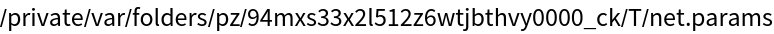Get the size of the parameter file:

 In:=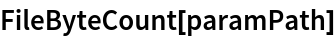Out=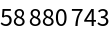The size is similar to the byte count of the resource object:

 In:=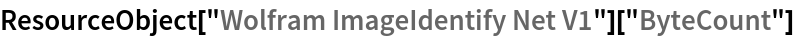Out=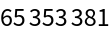## Requirements

Wolfram Language 11.1 (March 2017) or above

## Reference

• Wolfram Research